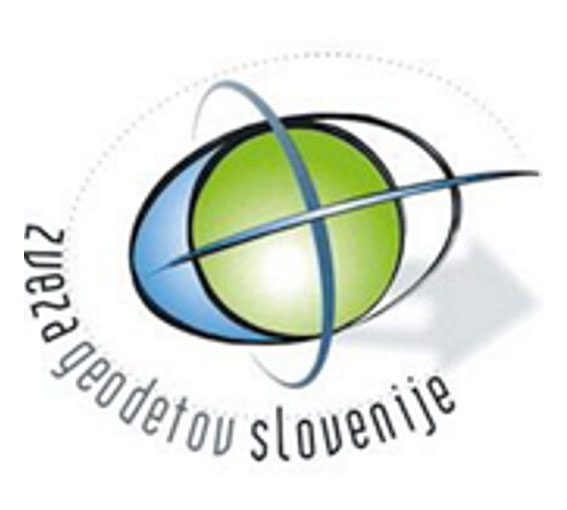### Paper

##### Robust determination of approximate coordinates in a horizontal geodetic network

Author(s):

Sandi Berk

Abstract:

A procedure of robust determination of the approximate coordinates of points in a horizontal geodetic network is presented. The primary aim is not accuracy but the reliability of the obtained coordinates. The motivation is to enable further processing according to the Gauss-Markov model. It is a linear mathematical model, thus the approximate values of unknowns are necessary input data. Disposable methods of determining coordinates of points and combinatorics of over-determined solutions dependent upon redundant observations are discussed. A geometrical approach is used, based on the fact that every point is an intersection of a pair of curves. The geometrical quality of each individual solution is evaluated from the intersection angle of both curves. A weight assigned to each solution is a function of that angle. Calculating coordinates is a successive procedure; each step assures the determination of one network point. The algorithm comprises searching for a typical solution among all solutions for an individual point. In order to avoid eventual gross errors, the procedure is based on robust statistics. The efficiency of three basic measures of location is tested on a practical example. The point is a generalization of mean, median, and mode i.e. the centre of mass, spatial median, and spatial mode. Gross errors are introduced into the network by using a Monte Carlo simulation.

Keywords:

approximate coordinates, horizontal geodetic network, measures of location, multivariate mode, poor solutions, robust statistics

Citation:

Sandi Berk (2010). Robustna določitev približnih koordinat v horizontalni geodetski mreži. | Robust determination of approximate coordinates in a horizontal geodetic network. Geodetski vestnik, 54 (1), 9-30. DOI: 10.15292/geodetski-vestnik.2010.01.009-030

ISSN: 0352-3551
EISSN: 1581-0267
COBISS: 3664386
UDK: (05) 532;556;626/628.6Publisher:
Association of Surveyors of Slovenia
Zemljemerska ulica 12, SI-1000 Ljubljana
E-mail: info@geodetski-vestnik.si